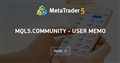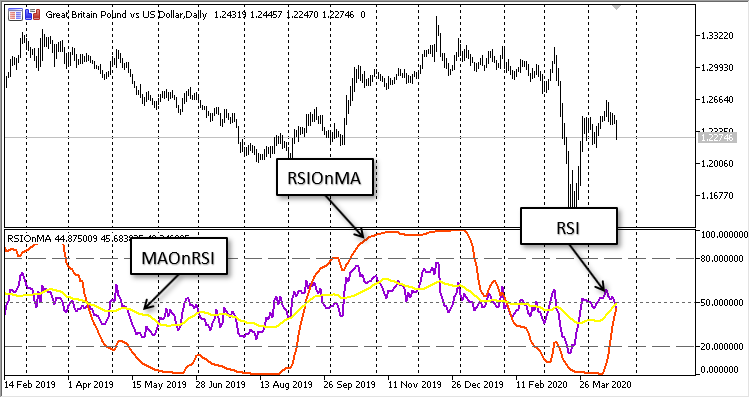# using iMa and iRSI

Hi

i want to use iMa and iRSI and compare values . but values are not true because of showing  iMa in chart windows and iRSI in Data window.

how can i use both of them in data window ?

Thanks.

1. You don't mean Data Window (control+d,) you mean separate window.
How To Ask Questions The Smart Way. 2004

2. Comparing values is non-sense. MA of price, has a range of 1.12345±, RSI [0…100]. If you could put MA of price (CI required) on an RSI window the MA would look flat at the bottom; useless. If you could put an RSI on a separate MA window, the RSI would almost always be off the top of the chart; useless.

3. If you want a MA of the RSI, open the Navigator (control+n) and drag the MA to the RSI window and set Apply to equal to Previous Indicators Data.

hi

exactly i want to do such as attached picture

but iMA is on the chart window and iRSI is on separate windows

i use

iMA(_Symbol,_Period,20,0,MODE_EMA,PRICE_CLOSE) ;

iRSI(_Symbol,_Period,14,PRICE_CLOSE);MQL5.community - User Memo
• www.mql5.com
You can now not only read articles and download MQL5 programs, but you can also join discussions on the forum, leave comments on articles and source codes, rate MQL5 programs and share your own developments in the Code Base, and even publish articles for a decent fee (see Become an Author at MQL5.com!). MQL5.com services are constantly...
Files:
Untitled.png  23 kb

Hi

i want to use iMa and iRSI and compare values . but values are not true because of showing  iMa in chart windows and iRSI in Data window.

how can i use both of them in data window ?

Thanks.

Check out this option: RSIOnMAOnRSI

### Indicator idea

Display three lines in one subwindow: RSI, RSI calculated for Moving Average and Moving Average calculated for RSIFigure: 1. Indicator 'RSIOnMAOnRSI'

Check out this option: RSIOnMAOnRSI

### Indicator idea

Display three lines in one subwindow: RSI, RSI calculated for Moving Average and Moving Average calculated for RSI

Figure: 1. Indicator 'RSThanks for